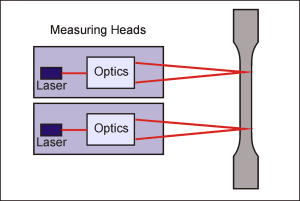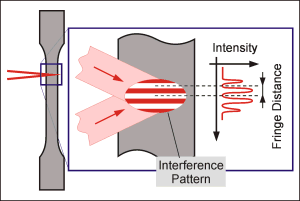Working Principle
 Overview Results Working PrincipleTwo coherent laser beams derived from a single source intersect at a fixed angle. The intersection volume of the two beams is positioned on the sample surface. The wavefronts of the two beams interfere in the intersection volume and form an interference pattern. The fringes of the pattern have a known distance depending on the wavelength of the laser and the angle between the two beams.For simplicity, assume that an element with a velocity component perpendicular to the center axis is moving through the intersection volume. The element will scatter light with its amplitude modulated by the local intensity contrast. The frequency of the modulation is proportional to the velocity of the element. Thus when recording the intensity signal the motion of elements within the observation area can be calculated. A typical sample with its natural roughness contains scattering elements everywhere on its surface which allows the measurement of the amount of material moving through the observation area. By simultaneous measurement at two points of the sample surface the relative motion between these two points can be determined. This allows the use of the measuring arrangement as an extensometer.